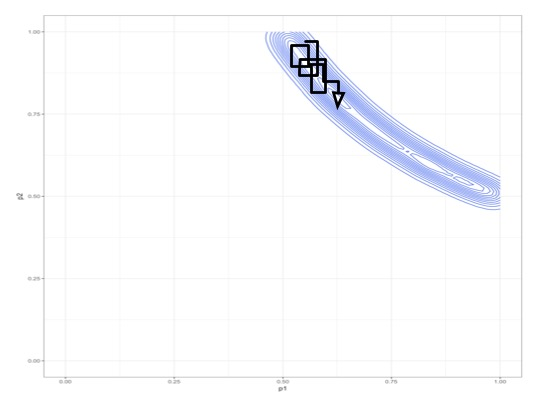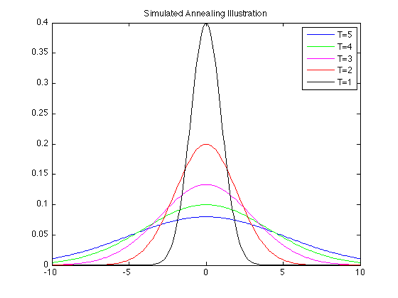# Motivation

Unidentifiably/weakly identifiable models create posterior distributions that are hard to sample from.• Note: many other standard MCMC schemes also struggle with this kind of problems (hit and run, Metropolis-Hastings, Langevin schemes, Hamiltonian, etc)

Solution: parallel tempering, the MCMC jackhammer

# Parallel tempering: annealingCombination of two ideas: “annealing” and “parallel chains”

• First idea: annealing
• let us start by assuming for simplicity the prior is uniform on a bounded region
• suppose a density has height $$h$$ at a point
• consider $$h^{0.0001}$$, note that this $$\approx 1$$, for both $$h=10$$ and $$h=0.1$$
• taking a small power of a positive number brings it closer to $$1$$
• let’s do that with every point in the landscape we seek to explore!

$\pi_{\beta}(x) = (\pi(x))^{\beta}$

where $$\beta$$ controls an interpolation between:

• the uniform distribution ($$\beta = 0$$, easy to sample from)
• the posterior distribution ($$\beta = 1$$, hard)

If the prior is defined over an unbounded set, generalize into: $\pi_{\beta}(x) = \text{prior}(x)(\text{likelihood}(x))^{\beta}$

Let us call $$\beta$$ the annealing parameter

Now we have several different “landscapes”, from smooth to rough. We will explore all of them simultaneously!

# Parallel tempering: swaps

• Let’s pick a sequence of annealing parameters, say $$(\beta_0, \dots, \beta_N) = (0, 1/N, 2/N, \dots, 1)$$
• Second idea: parallel tempering
• Run several interacting MCMC chains in parallel (each say based on slice sampling) for each landscape $$\pi_i(x) = \pi_{\beta_i}$$ including the easy-to-explore landscape ($$i=0$$ where $$\beta = 0$$) and the landscape of interest ($$i=N$$ where $$\beta = 1$$)
• Idea formalized using an augmented distribution $\tilde \pi(x_0, x_1, \dots, x_N) = \pi_0(x_0) \pi_1(x_1) \dots \pi_N(x_N)$
• This ensemble of chains provides us with samples from the high dimensional distribution $$\tilde \pi(x_0, x_1, \dots, x_N)$$
• At the end, only keep the states in $$x_N$$, i.e. those corresponding to $$\beta = 1$$ (the posterior)
• But use the other ones to propose swaps between pairs of chains to allow “jumps” across the state space
• Swap are accepted or rejected according to the Metropolis rule from earlier today (coming up: can you derive the acceptance probability?)
• Visualization of the algorithm: pt-algo.pdf

### Poll: what is the swap acceptance ratio?

Suppose you just proposed a swap between chain $$i$$ and $$i+1$$

1. One
2. $$(\pi_i(x_{i}) \pi_{i+1}(x_{i+1})) / (\pi_i(x_{i+1}) \pi_{i+1}(x_{i}))$$
3. $$(\pi_i(x_{i+1}) \pi_{i+1}(x_{i})) / (\pi_i(x_{i}) \pi_{i+1}(x_{i+1}))$$
4. $$\pi_i(x_{i+1}) / \pi_{i+1}(x_{i})$$
5. $$\pi_{i+1}(x_{i}) / \pi_i(x_{i+1})$$

# Swap acceptance ratio

Let $$x = (x_0, x_1, \dots, x_N)$$ denote the current state, and the proposed state is $$x' = (x_0, x_1, \dots, x_{i-1}, \color{blue}{x_{i+1}}, \color{red}{x_i}, x_{i+2}, \dots, x_N)$$

Note: the proposal is symmetric, hence the ratio ignores the proposal and only involves the ratio of augmented target distributions:

\begin{align*} \frac{\tilde \pi(x')}{\tilde \pi(x)} &= \frac{\pi_0(x_0) \pi_1(x_1) \dots \pi_{i-1}(x_{i-1}) \color{blue}{\pi_i(x_{i+1})} \color{red}{\pi_{i+1}(x_i)} \pi_{i+2}(x_{i+2}) \dots \pi_N(x_N)}{\pi_0(x_0) \pi_1(x_1) \dots \pi_{i-1}(x_{i-1}) \color{blue}{\pi_i(x_{i})} \color{red}{\pi_{i+1}(x_{i+1})} \pi_{i+2}(x_{i+2}) \dots \pi_N(x_N)} \\ &= \frac{\color{blue}{\pi_i(x_{i+1})} \color{red}{\pi_{i+1}(x_i)}}{\color{blue}{\pi_i(x_{i})} \color{red}{\pi_{i+1}(x_{i+1})}}. \end{align*}

# A law of large numbers

We now have all the ingredients to demonstrate how the “MCMC methodology game” can be applied to get a law of large numbers for the parallel tempering algorithm.

1. The swap move is a MH move and hence is $$\pi$$-invariant
2. We assume the “local exploration kernels” are $$\pi$$-invariant and irreducible
3. Hence using either mixture of alternation of the “local exploration steps” and “communication steps” yields a $$\pi$$-invariant and irreducible sampler
4. Hence, we have a law of large numebers

Interesting twist coming up soon: deciding which of mixture or alternation to use can have a huge effect in the context of parallel tempering!

# Non-reversible parallel tempering

• You may need many parallel chains to be able to swap frequently
• Reversible parallel tempering gets really slow in this context
• Ongoing work in my research group: non-reversible parallel tempering avoid this issue

Parallel Tempering: Reversible (top) versus non-reversible (bottom)